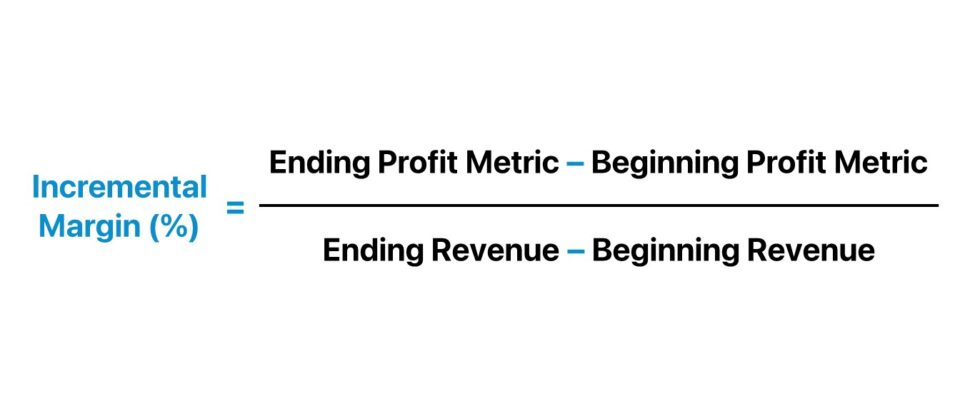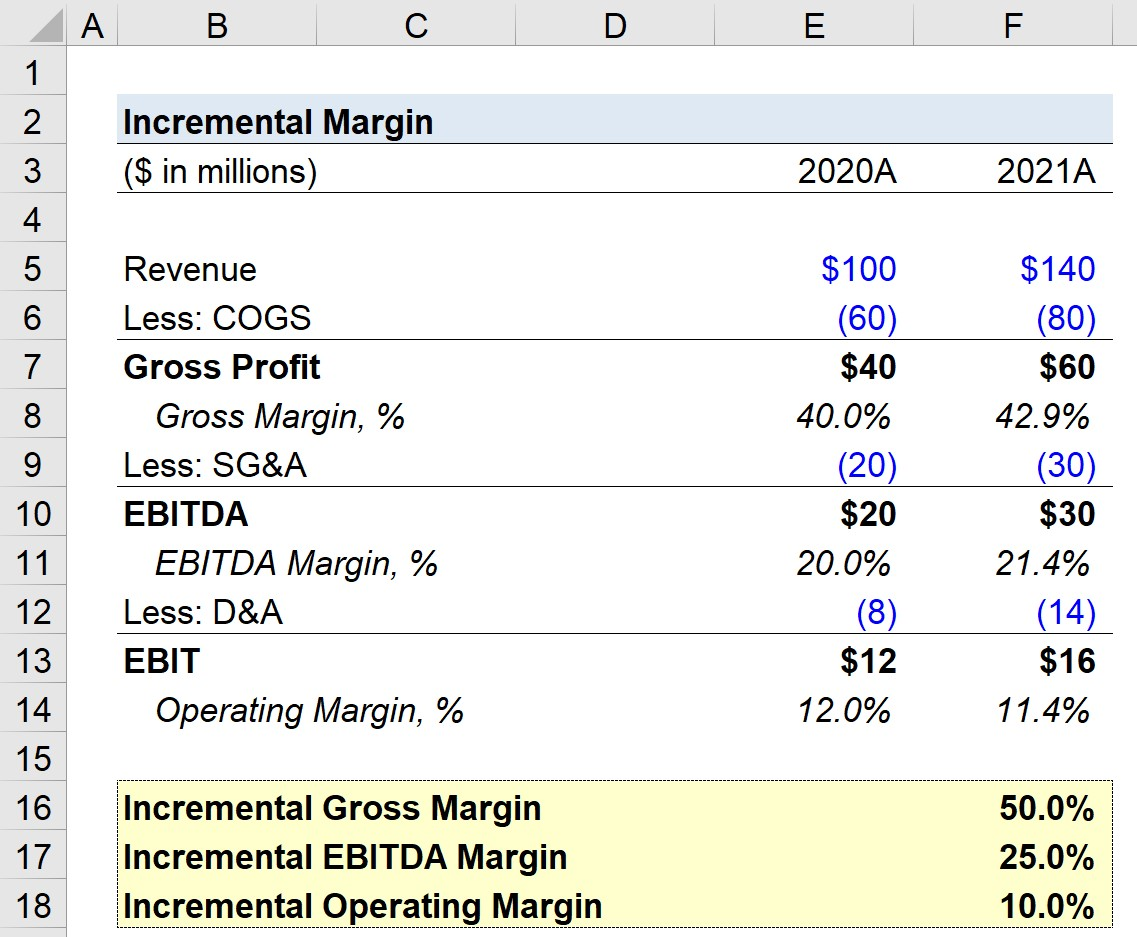Welcome to Wall Street Prep! Use code at checkout for 15% off.# Incremental Margin

Guide to Understanding the Incremental Margin## How to Calculate Incremental Margin?

A profit margin measures the percentage of a company’s net revenue remaining once certain expenses have been deducted.

Formulaically, most profit margin metrics are a ratio between a profitability metric and revenue, i.e. the “top line” of the income statement.

Profit Margin (%) = Profit Metric ÷ Revenue

By comparing the profit metric to revenue, one can estimate a company’s profitability and identify its cost structure, i.e. where most of the company’s spending is allocated.

The profit margins of a given company can be compared to its industry peers to determine if the company operates more efficiently (or less efficiently) relative to its competitors.

The most common profit margin metrics are the following:

Profit Margin Formula Description
Gross Margin Gross Margin (%) = Gross Profit ÷ Revenue
• The gross profit is the difference between revenue and cost of goods sold (COGS).
• The gross margin (%) is the ratio between gross profit and revenue in the coinciding period, expressed as a percentage.
Operating Margin Operating Margin (%) = EBIT ÷ Revenue
• The operating income (or “EBIT”) is the residual profits after COGS and operating expenses, such as SG&A and R&D were deducted.
• The operating margin (%) is the ratio between the operating income (EBIT) of a company and the revenue generated in the period, expressed as a percentage.
• The operating profit margin establishes a relationship between EBIT and revenue to determine the profitability before non-operating expenses, such as interest expense and taxes.
EBITDA Margin EBITDA Margin (%) = EBITDA ÷ Revenue
• EBITDA is a non-GAAP measure of operating performance intended to measure the core profitability of a company on a normalized basis.
• The EBITDA margin (%) is the ratio between a company’s EBITDA and revenue, expressed as a percentage.
Net Profit Margin Net Profit Margin (%) = Net Income ÷ Revenue
• The net income metric is the difference between pre-tax income (EBT) and taxes.
• The net profit margin (%) measures the percentage of earnings remaining once all costs and expenses were deducted from the revenue generated in the period.
• The net margin reflects the earnings, upon deducting all costs, that belong to the company per dollar of revenue produced.

Profit margins can be informative as standalone metrics, yet another method to analyzing the performance of companies is to calculate the incremental margin, which shows the direction that profit margins are moving as a result of changes in revenue.

## Incremental Margin Formula

The formula for calculating the incremental margin is as follows.

Incremental Margin (%) = (Ending Profit Metric Beginning Profit Metric) ÷ (Ending Revenue Beginning Revenue)

If, for example, we’re calculating the incremental EBITDA margin, we’ll replace the “Profit Metric” with “EBITDA”.

Incremental EBITDA Margin (%) = (Ending EBITDA Beginning EBITDA) ÷ (Ending Revenue Beginning Revenue)

## How to Interpret Incremental Margin?

In particular, the incremental margin is important for cyclical companies, where performance is tied to an external factor such as the current economic conditions.

For cyclical industries, such as manufacturing and industrials, strong margins are crucial because it reflects that a company can capitalize at the top of the cycle and manage its margins in a down cycle, where demand is reduced and margins are pressured.

Companies that have exhibited cyclical performance in the past must take into account their margin “cushion” because it determines the buffer it has if the economy were to undergo a contraction or enter a recession.

The incremental margin metric is also closely tied to the concept of operating leverage, as a company’s cost structure – i.e. proportion of fixed vs. variable costs – largely determines how its profit margins uphold throughout different economic cycles.

## Incremental Margin Calculator

We’ll now move to a modeling exercise, which you can access by filling out the form below.Submitting...

## Incremental Margin Calculation Example

Suppose we’re tasked with calculating the incremental margin for a company from 2020 to 2021.

The financials of our hypothetical company are shown below, along with the associated profit margins.

Operating Assumptions
(\$ in millions) 2020A 2021A
Revenue \$100 million \$140 million
Less: COGS (60 million) (80 million)
Gross Profit \$40 million \$60 million
Gross Margin, % 40.0% 42.9%
Less: SG&A (20 million) (30 million)
EBITDA \$20 million \$30 million
EBITDA Margin, % 20.0% 21.4%
Less: D&A (8 million) (14 million)
EBIT \$12 million \$16 million
Operating Margin, % 12.0% 11.4%

From 2020 to 2021, we can see that the gross margin expanded from 40.0% to 42.9%, while the EBITDA margin expanded from 20.0% to 21.4%.

However, the operating margin of our company, contrary to the gross margin and EBITDA margin, declined from 12.0% to 11.4%.

Our hypothetical company’s gross profit grew by \$20 million year-over-year (YoY), while revenue increased from \$100 million to \$140 million.

If we focus solely on the year-over-year change – i.e. the incremental difference – the incremental gross margin is \$20 million divided by \$40 million, which comes out to 50.0%.

Since we have all the necessary inputs to calculate the incremental margins, we’ll apply the formula for each profit metric.

• Incremental Gross Margin = (\$60 million – \$40 million) ÷ (\$140 million – \$100 million) = 50.0%
• Incremental EBITDA Margin = (\$30 million – \$20 million) ÷ (\$140 million – \$100 million) = 25.0%
• Incremental Operating Margin = (\$16 million – \$12 million) ÷ (\$140 million – \$100 million) = 10.0%Step-by-Step Online Course

### Learn Buy-Side (Hedge Fund) Modeling

Led by a former hedge fund PM (Maverick, Citadel, DE Shaw, Schonfeld), this program begins where financial modeling training ends — with a deep-dive into how buy-side analysts build financial models to make key investment decisions.